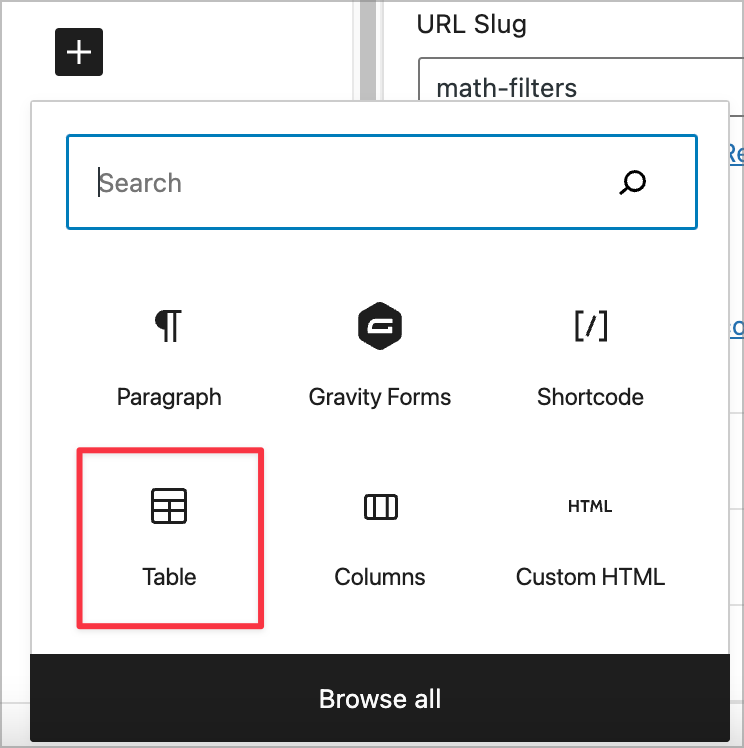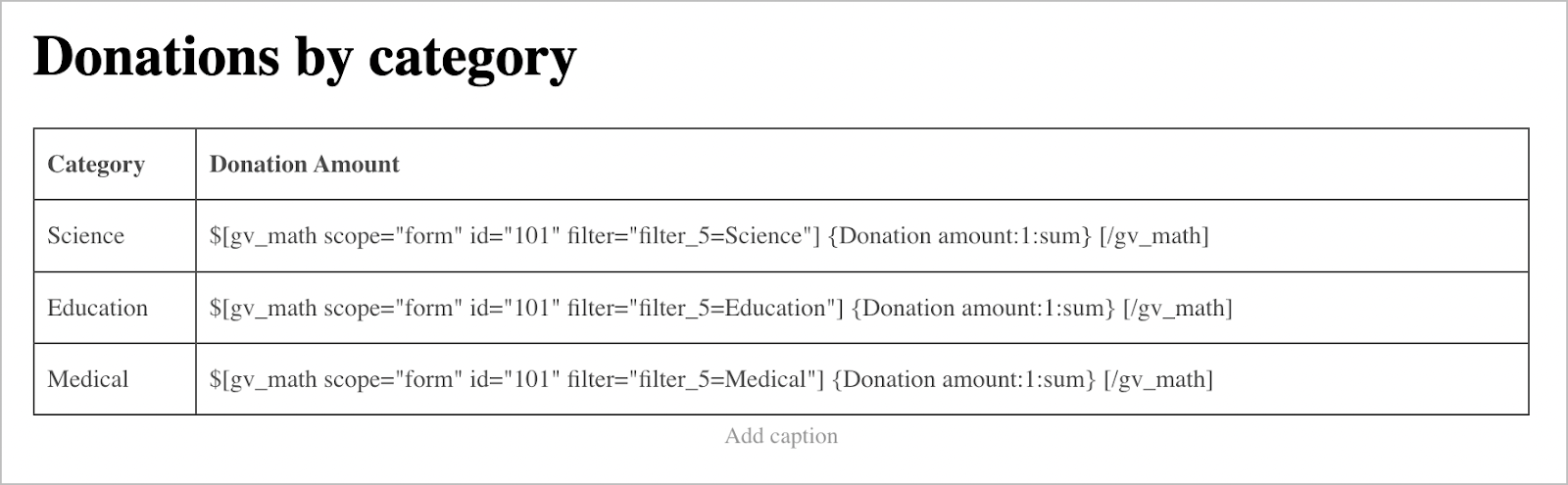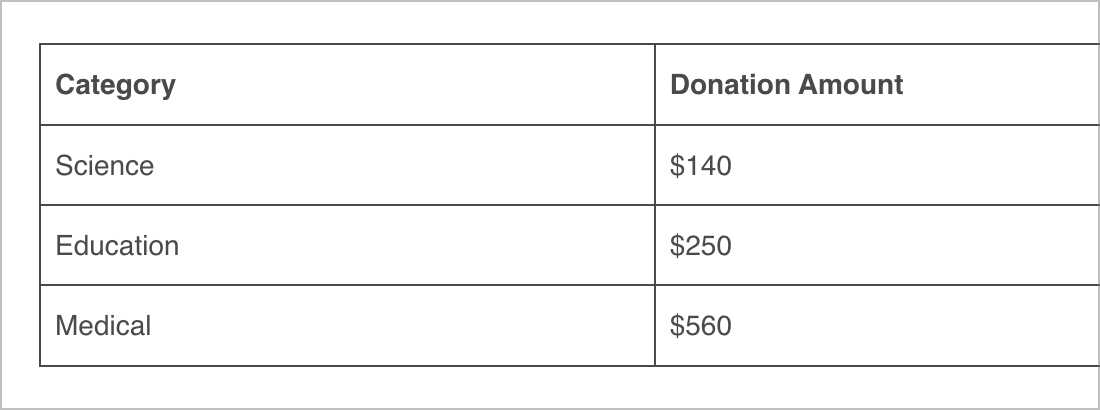# How to Display Summaries Using [gravitymath]

You may want to group Gravity Forms entries by a specific field value and then perform math operations on entries within those groups.

Here’s an example: You have a donation form on your website allowing users to donate towards different causes (e.g., Science, Education, and Medical). Now you want to calculate the total amount donated towards each cause.

This is possible using the `[gravitymath]` "filter" parameter.## Constructing the Shortcodes

As you can see, our donation form allows users to enter a custom donation amount and then choose which cause they want their money to go towards (Science, Education or Medical).

Instead of creating a View that displays each donation, we’re going to create a table that summarizes the total amount donated towards each cause.

There’s no way to do this automatically in GravityView, so we’ll need to manually construct a `[gravitymath]` shortcode for each donation category using the `filter` parameter:

Science: `[gravitymath scope="form" id="101" filter="filter_5=Science"] {Donation amount:1:sum} [/gravitymath]`

Education: `[gravitymath scope="form" id="101" filter="filter_5=Education"] {Donation amount:1:sum} [/gravitymath]`

Medical: `[gravitymath scope="form" id="101" filter="filter_5=Medical"] {Donation amount:1:sum} [/gravitymath]`

We’re using `scope="form"` because we’re only working with entries submitted through our donation form (with ID “101”). Next, we’re filtering by the category using the `filter` parameter `filter="filter_5=Science"` (“5” is the ID of the field we want to filter by).

Inside the shortcode, we place the merge tag for the field we want to sum. If we wanted to count the number of entries instead of summing them, we could do that using the `count` modifier.

## Creating the Summary Table

To display these calculations in a table, we’ll edit our page and add a new “Table” block.We’ll create a table with two columns and four rows. We’ll add the donation categories to the column on the left, and then we’ll paste our `[gravitymath]` shortcodes into the column on the right.Here’s what our summary table looks like on the front end:When you receive new donations, your table will update to reflect the new amounts.

## Filtering by User

You may want to group entries by the user who created them and then perform math calculations on those entries. That’s also possible using the `[gravitymath]` shortcode and the `filter` parameter. Here’s an example:

`[gravitymath scope="form" id="101" filter="filter_created_by=1"] {Donation amount:1:sum} [/gravitymath]`

In this case, the “1” is the user ID of a specific user on your website.

`[gravitymath scope="form" id="101" filter="filter_5=Science&filter_created_by=1"] {Donation amount:1:sum} [/gravitymath]`